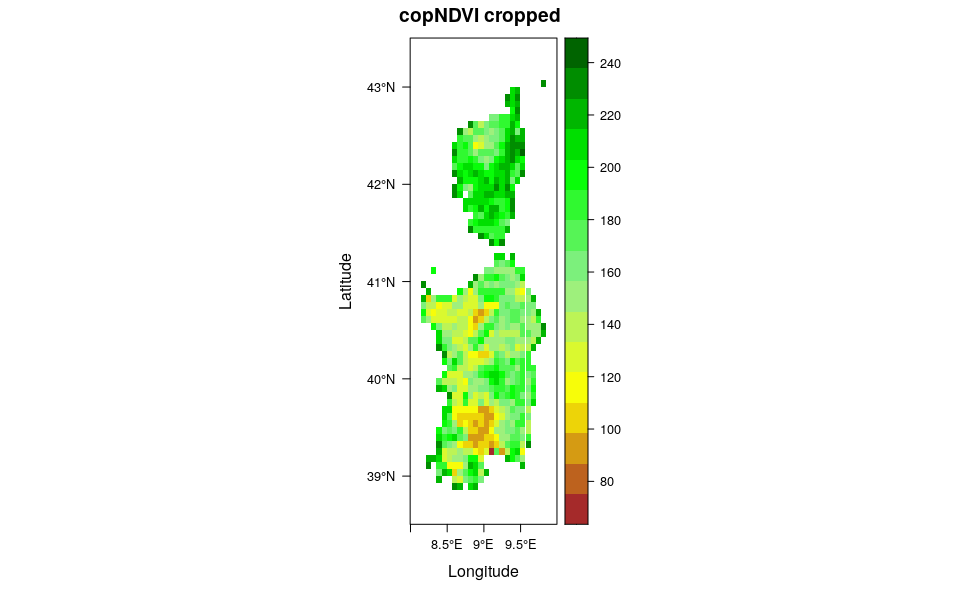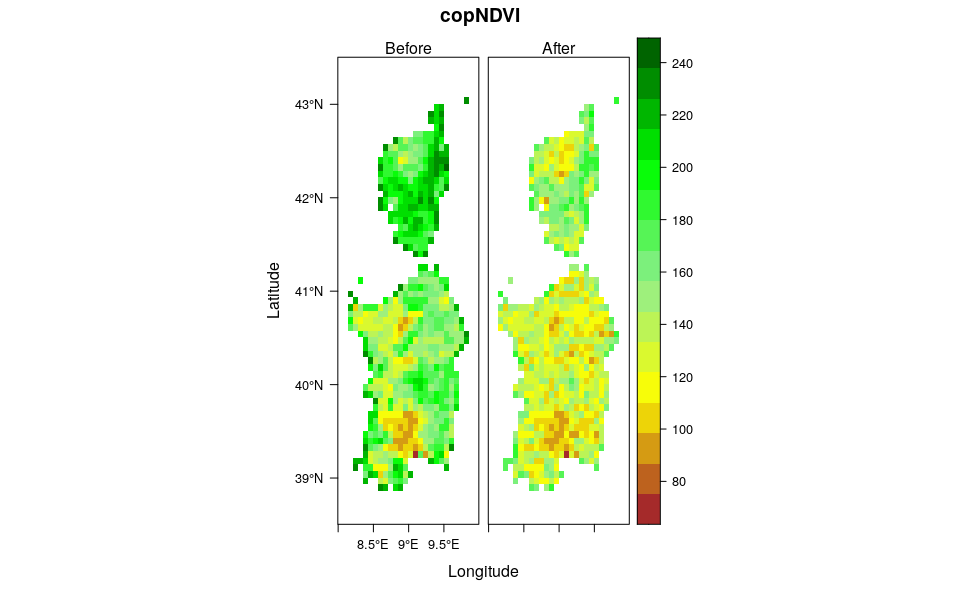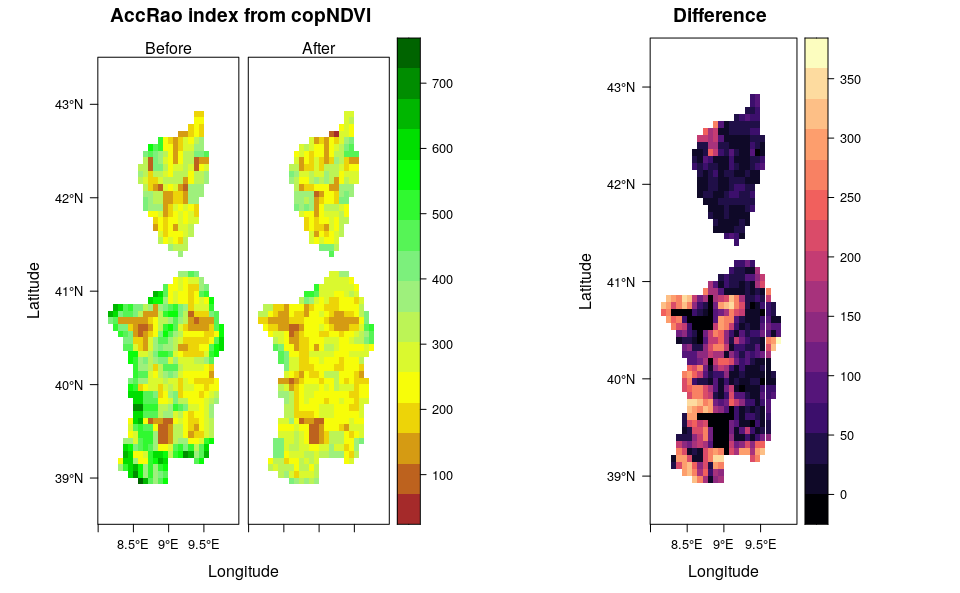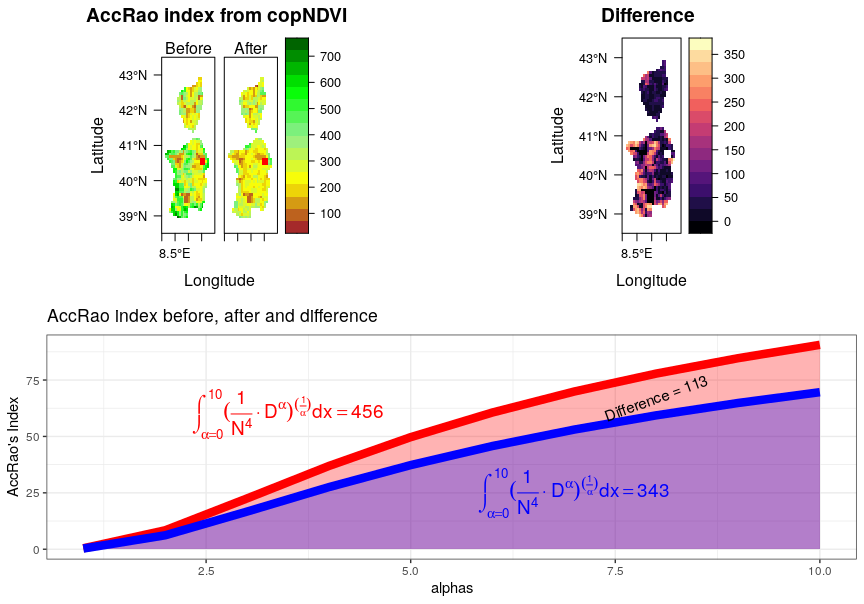# Rasterdiv: Derive the accumulation Rao’s index.

#Required packages
require(rasterdiv)
require(rasterVis)
require(ggplot2)
require(gridExtra)

This vignette uses rasterdiv to calculate the accumulation function (integral) of Rao values calculated using a range of alphas. The rationale of using the accumulation function to report values of the parametric Rao’s index it is that it may be hard to choose an single alpha level and thus integrating over a range of alphas may be more convenient.

## Overview

A RasterLayer called copNDVI is loaded together with the package rasterdiv. copNDVI is a 8-bit raster, meaning that pixel values range from 0 to 255.

## Reclassify NDVI

Pixels with values 253, 254 and 255 (water) will be set as NA’s.

copNDVI <- raster::reclassify(copNDVI, cbind(252,255, NA), right=TRUE)

## Resample NDVI to a coarser resolution

For the sake of speeding up the calculation, copNDVI will be cropped on Sardinia and Corsica in the Mediterranean Sea.

ndvi.before <- raster::crop(copNDVI, extent(8,10,38.5,43.5))
#Set float numbers as integers to further speed up the calculation
storage.mode(ndvi.before[]) = "integer"
col.ndvi <- colorRampPalette(c('brown', 'yellow', 'lightgreen','green', "darkgreen"))
levelplot(ndvi.before,layout=c(1,1), margin=FALSE, col.regions=col.ndvi,main="copNDVI cropped")## Simulating a decrease of high value on NDVI (for example due to widespread forest fires)

Pixels with NDVI values higher than 150 will be decreased using values from a normal distribution centered on 50 with a standard deviation of 5.

ndvi.after <- ndvi.before
ndvi.after[ndvi.after>=150] <- ndvi.after[ndvi.after>=150] - as.integer(rnorm(length(ndvi.after[ndvi.after>=150]),mean=50,sd=5))
#Set float numbers as integers to further speed up the calculation
storage.mode(ndvi.after[]) = "integer"
levelplot(stack(ndvi.before,ndvi.after),layout=c(2,1), margin=FALSE,col.regions=col.ndvi,main="copNDVI", names.attr=c("Before", "After"))## Compute the accumulation Rao’s index

#The accumulation Rao's index (accRao) will be calculated for the two images and for each pixel using alphas ranging from 1 to 10.
accrao.before <- accRao(alphas=1:10, x=ndvi.before, dist_m="euclidean", window=3, method="classic", rasterAUC=TRUE, na.tolerance=0.4, np=1)
accrao.after <- accRao(alphas=1:10, x=ndvi.after, dist_m="euclidean", window=3, method="classic", rasterAUC=TRUE, na.tolerance=0.4, np=1)

#The absolute difference between before and after can now be calculated
accrao.diff <- abs(accrao.after - accrao.before)

## Visualise the difference

l1 <- levelplot(stack(accrao.before,accrao.after),as.table = T, layout=c(0,2,1), margin=FALSE,col.regions=col.ndvi, names.attr=c("Before", "After"),main="AccRao index from copNDVI")
l2 <- levelplot(accrao.diff, layout=c(1,1), margin=FALSE, main="Difference")

grid.arrange(l1,l2, layout_matrix = rbind(c(1,2)))We’ll now show how the accumulation function of the parametric Rao’s index (accRao) is calculated in rasterdiv, focussing on a single moving window (9 pixels) from the NDVI before and after.

First we will extract a 3x3 pixel area from the two rasters

ndvi.t0 <- ndvi.before[41:43, 21:23,drop=FALSE]
ndvi.t1 <- ndvi.after[41:43, 21:23,drop=FALSE]
alphas = 1:10 #set the alpha interval over which to integrate Rao's index
N = 3^2 #and set the number of pixels in the selected window

Now we need a simple function that calculates parameteric Rao’s Index over a vector of NDVI values

RaoFx <- function(alpha,N,D) {( sum((1/(N^4)) * D^alpha )*2)^(1/alpha)}

Rao’s index can thus be calculated for the two vectors of NDVI values at t0 and t1 and for alpha ranging from 1 to 10

rao.t0 <- sapply(alphas, function(a) {RaoFx(alpha=a, N=N,D=as.vector(ndvi.t0))})
rao.t1 <- sapply(alphas, function(a) {RaoFx(alpha=a, N=N,D=as.vector(ndvi.t1))})

The next step is the integration of the vector of Rao’s values at t0 and t1. Before we can perform the integration, we need to approximate a function which interpolates the values of Rao’s index at each alpha.

#to
accrao.t0f <- approxfun(x=alphas,y=rao.t0)
accrao.t0 <- integrate(accrao.t0f, lower=1,upper=10, subdivisions = 500)
print(accrao.t0)
#t1
accrao.t1f <- approxfun(x=alphas,y=rao.t1)
accrao.t1 <- integrate(accrao.t1f, lower=1,upper=10, subdivisions = 500)
print(accrao.t1)

Now that we have the values of the two integral we visualize the differences between the two accumulation functions representing them as area under the curve.

accrao.df <- cbind.data.frame(alphas,rao.t0,rao.t1,alphas1=rep(0,10))
g1 <- ggplot(accrao.df,aes(x=alphas,y=rao.t0)) +
ylab("AccRao's Index") +
geom_line(col="red",lwd=3) +
geom_area(data=accrao.df,aes(x=alphas,y=rao.t0),fill="red",alpha=0.3,inherit.aes=FALSE) +
geom_area(data=accrao.df,aes(x=alphas,y=rao.t1),fill="blue",alpha=0.3,inherit.aes=FALSE) +
geom_line(data=accrao.df,aes(x=alphas,y=rao.t1),col="blue",lwd=3,inherit.aes=FALSE) +
geom_text(data=cbind.data.frame(x=3.5,y=60),aes(x=x,y=y),label=expression(integral((frac(1, N^4) %.% D^alpha)^(frac(1,alpha)) * dx == 456, alpha==0, 10)),col="red",cex=5,inherit.aes=FALSE) +
geom_text(data=cbind.data.frame(x=7,y=25),aes(x=x,y=y),label=expression(integral((frac(1, N^4) %.% D^alpha)^(frac(1,alpha)) * dx == 343, alpha==0, 10)),col="blue",cex=5,inherit.aes=FALSE) +
geom_text(data=cbind.data.frame(x=8,y=67),aes(x=x,y=y),label="Difference = 113",col="black",cex=4,angle=20,inherit.aes=FALSE) +
ggtitle("AccRao index before, after and difference") +
theme_bw()

#Everything in one plot, the red and white squares overlayed on the rasters represent the moving window selected for the exercise.
l1 <- l1+levelplot(ndvi.t0,col.regions="red")
l2 <- l2+levelplot(ndvi.t0,col.regions="white")
grid.arrange(l1,l2,g1, layout_matrix = rbind(c(1,2),c(3,3)))